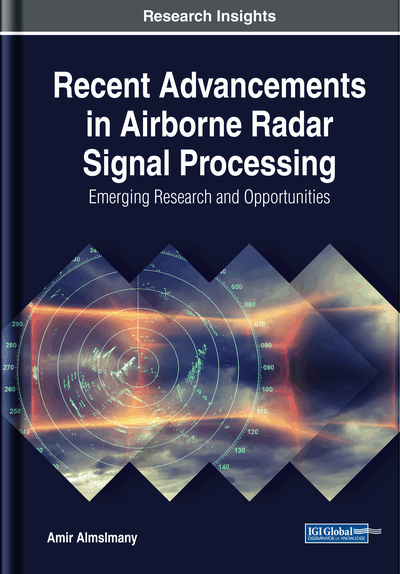# Target Detection

DOI: 10.4018/978-1-5225-5436-3.ch002
Available
\$33.75
List Price: \$37.50
10% Discount:-\$3.75
TOTAL SAVINGS: \$3.75

## Abstract

Target detection means to illuminate the target and then calculate its parameters, such a range, speed, azimuth, and altitude. The target range can be obtained using one of several methods: by calculating the time between the detected target echo and the transmitted wave, then calculating the difference in frequency between the received echo and the transmitted wave in the case of linear frequency modulation, and finally calculating the differential phase of the double detection of an echo obtained using two transmissions of different frequencies.
Chapter Preview
Top

## Range Detection

The radar range equation can be written as follows (mahfaza, 2004):

(1) whereis the transmitted peak power,,are the transmitter and the receiver gain, respectively.is the wavelength andis the radar cross section area, andis the Boltzmann’s constant(Watt*sec/°Kelvin), andis the temperature 290 K, andis the receiver band width, andis the receiver noise Figure, andis the loss factor.

Figure 1 is plotted to show the relation between the radar equation and the target detection process. In airborne radar, the range processing is the same as the ground radars, but it suffers from more problems, compared with the ground radars, these problems must be compensated in order to get high probability of detection.

The main important problems are the power loss due to targets range and many other reasons, the limited input dynamic range for radar receiver, the range migration problems (range walk, range curvature, acceleration), and Doppler ambiguity estimation, in the following section we will discuss these problems in details.

## Complete Chapter List

Search this Book:
Reset